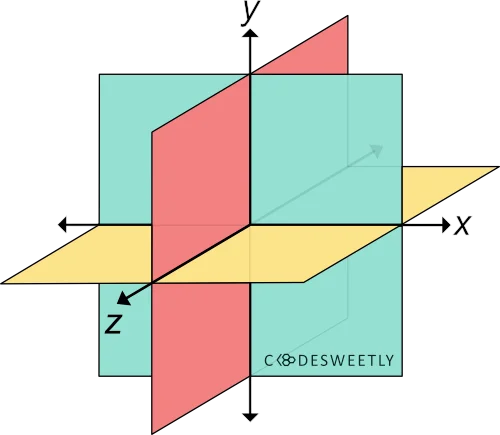# CSS scale3d() Function – How to Resize Elements in 3D

scale3d() transforms an element by resizing (scaling) it three-dimensionally from a fixed point along the x-, y-, and z-axis.A three-dimensional Cartesian coordinate system showing the X-, Y-, and Z-axis
note
• "Transform origin" is the fixed point from which the computer scales an element.
• You can define your element's fixed point using the CSS transform-origin property. But the default is center.

## Syntax of the CSS scale3d() Function​

scale3d() accepts three arguments. Here is the syntax:

element {  transform: scale3d(x, y, z);}

The x, y, and z arguments are numbers specifying the x-, y-, and z-coordinates. The coordinates are the axis along which browsers will scale the element.

note

Whenever you include scaling or zooming animations in your app, provide users an option to turn off animations. This option is necessary because scaling and zooming animations cause accessibility issues.

## Examples of the CSS scale3d() Function​

Below are some examples of how the CSS scale3d() function works.

### How to use scale3d() with CSS perspective() and rotateX() functions​

img {  transform: perspective(370px) scale3d(1, 1, 5) rotateX(17deg);  width: 80%;}

Try Editing It

Here's what we did in the snippet above:

1. We used the perspective() function to define a 370px distance between the user and the z=0 plane.
2. The scale3d() function specifies a scale factor of 1, 1, and 5 for the image along the x-, y-, and z-axis.
3. We used the rotateX() function to rotate the image seventeen-degree (17⁰) around the x-axis.
info
• A scale factor of 1 will apply no scaling effect on the element.
• List perspective() first whenever you chain it with other CSS transform functions. Otherwise, browsers might transform the selected element incorrectly.
• List the scale3d() function before rotateX(). Otherwise, the browser will not scale the element.

### How to scale elements three-dimensionally​

img {  width: 40%;}.second-image {  transform: scale3d(5, 3, 0.05);  transform-origin: top left;}

Try Editing It

We used the scale3d() function to specify a scale factor of 5, 3, and 0.05 for the image along the x-, y-, and z-axis.

## Overview​

This article discussed what a CSS scale3d() function is. We also used examples to see how it works.